# pgtual and inverted 6. The charge on each plate of a parallel-plate capacitor is 20 uC. The capacitance of the capacitor is C. 1 HF 10-6 F. 12) What is the magnitude of the electric field betwee...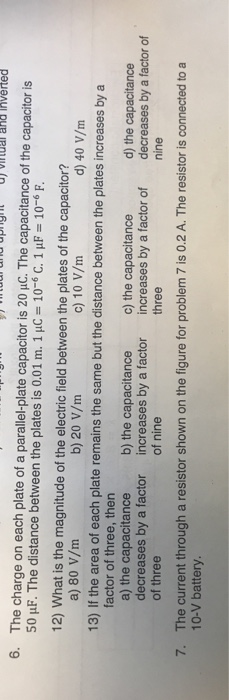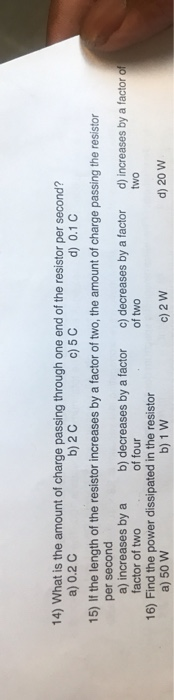pgtual and inverted 6. The charge on each plate of a parallel-plate capacitor is 20 uC. The capacitance of the capacitor is C. 1 HF 10-6 F. 12) What is the magnitude of the electric field between the plates of the capacitor? a) 80 V/m 13) If the area of each plate remains the same but the distance between the plates increases by a factor of three, thern a) the capacitance b) the capacitance c) the capacitance decreases by a factor increases by a factor increases by a factor of decreases by a factor of of three b) 20 V/m c) 10 V/m d) 40 V/m d) the capacitance three nine of nine 7. The current through a resistor shown on the figure for problem 7 is 0.2 A. The resistor is connected toa 10-V battery.
14) What is the amount of charge passing through one end of the resistor per second? a) 0.2 C b) 2 C c) 5 C d) 0.1 C 15) If the length of the resistor increases by a factor of two, the amount of charge passing the resistor per second a) increases by a b) decreases by a factor c) decreases by a factor d) increases by a factor of factor of two 16) Find the power dissipated in the resistor a) 50 W 8 of four of two two b) 1 W c) 2 W d) 20 W

Dear student,

Find this solution.if any issue with that don't forget to write in comment section.I will rectify them as soon as possible.

If you find the solution helpful and kindly RATE THE ANSWER it would be appreciated.

Your rating is important to me.

Thanks for asking..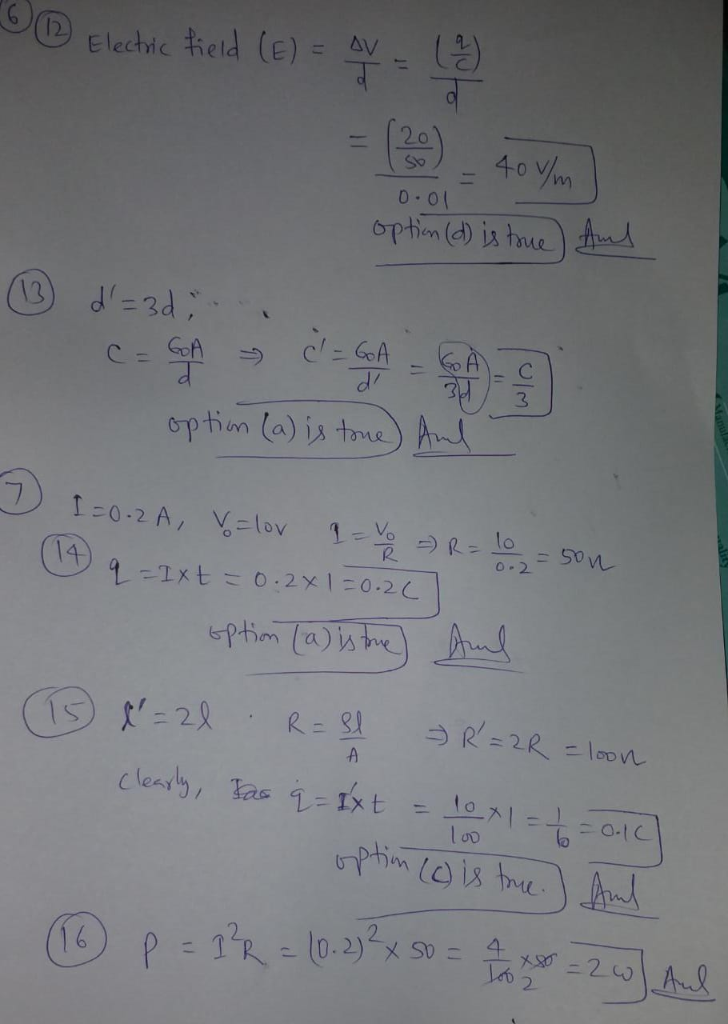#### Earn Coin

Coins can be redeemed for fabulous gifts.

Similar Homework Help Questions
• ### of an electromagnetic wave propagating in a vacuum is 65 x 10 Hz. See the table for problem 1 1) To what part of the electromagnetic spectrum does this wave a) blue light 2) The electromag a)per...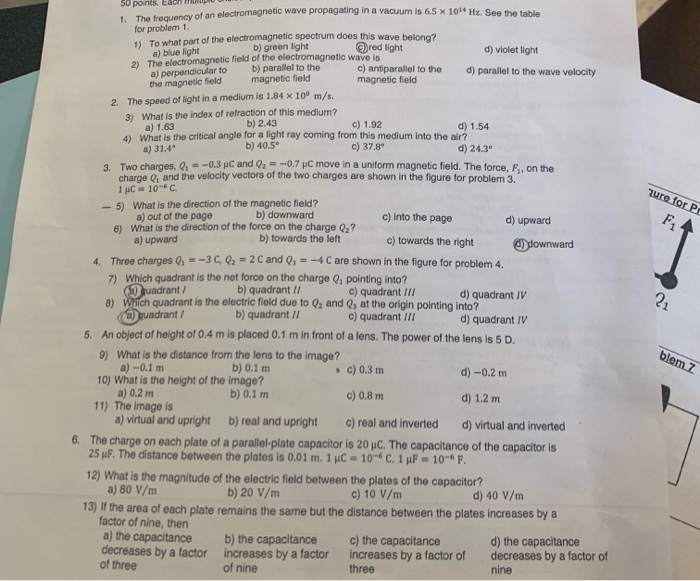of an electromagnetic wave propagating in a vacuum is 65 x 10 Hz. See the table for problem 1 1) To what part of the electromagnetic spectrum does this wave a) blue light 2) The electromag a)perpendicular to b) parallel to the the magnetic field b) green light netic field of the electromagnetic wave is d) violet light c) antiparallel to the d) paralel to the wave velocity magnetic field magnetic field The speed of light in a medium is...

• ### A parallel-plate capacitor has charge of magnitude 8.40 µC on each plate and capacitance 3.60 µF...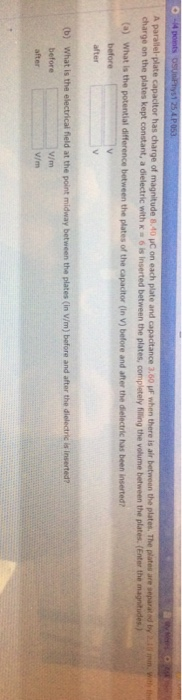A parallel-plate capacitor has charge of magnitude 8.40 µC on each plate and capacitance 3.60 µF when there is air between the plates. The plates are separated by 2.10 mm. With the charge on the plates kept constant, a dielectric with κ = 6 is inserted between the plates, completely filling the volume between the plates. (Enter the magnitudes.) (a) What is the potential difference between the plates of the capacitor (in V) before and after the dielectric has been...

• ### Question 4 1 pts A parallel-plate capacitor of capacitance C has the area of plates A...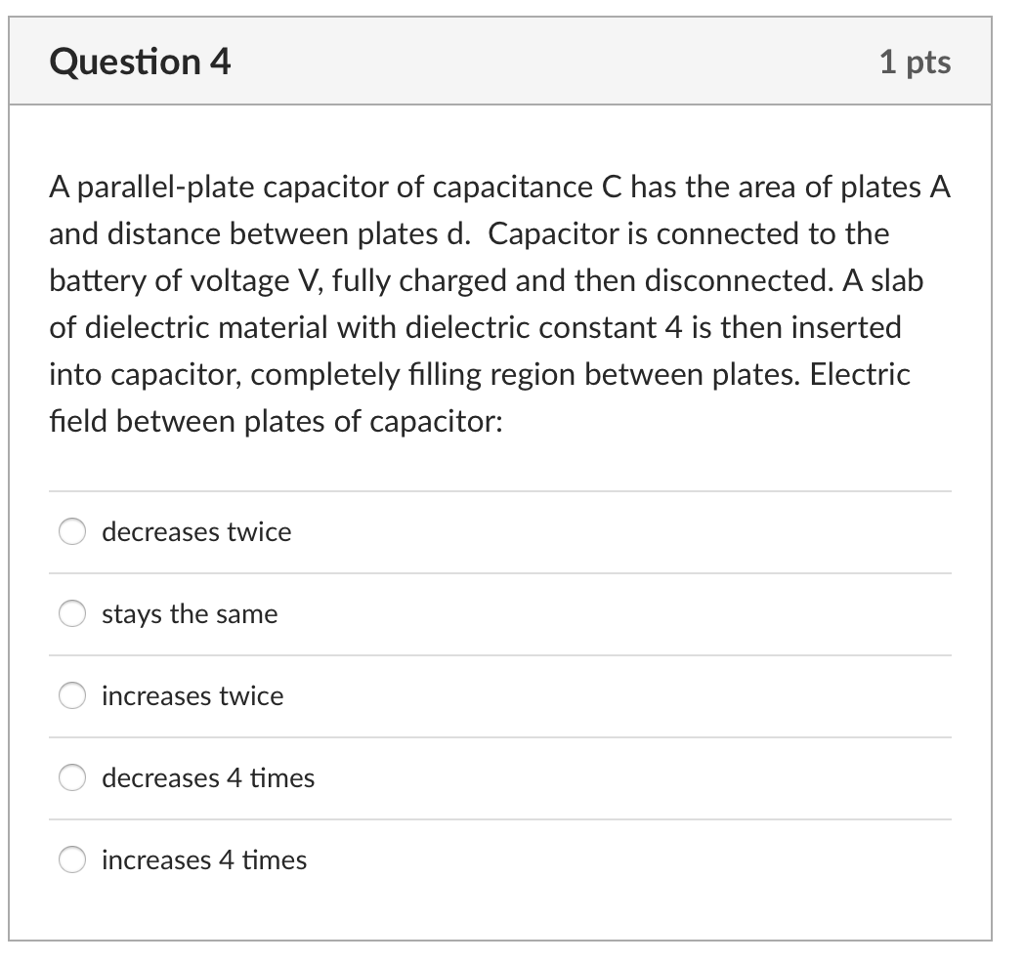Question 4 1 pts A parallel-plate capacitor of capacitance C has the area of plates A and distance between plates d. Capacitor is connected to the battery of voltage V, fully charged and then disconnected. A slab of dielectric material with dielectric constant 4 is then inserted into capacitor, completely filling region between plates. Electric field between plates of capacitor: decreases twice stays the same increases twice decreases 4 times increases 4 times

• ### Question 4 1 pts A parallel-plate capacitor of capacitance C has the area of plates A...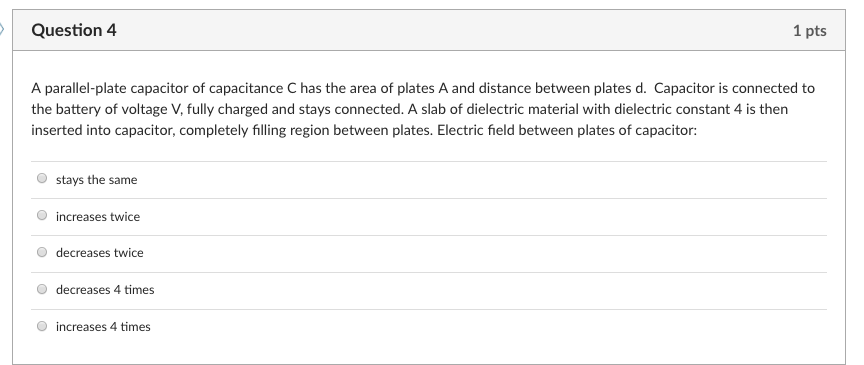Question 4 1 pts A parallel-plate capacitor of capacitance C has the area of plates A and distance between plates d. Capacitor is connected to the battery of voltage V, fully charged and stays connected. A slab of dielectric material with dielectric constant 4 is then inserted into capacitor, completely filling region between plates. Electric field between plates of capacitor: O stays the same O increases twice O decreases twice O decreases 4 times O increases 4 times

• ### The magnitude of the charge on each plate of a parallel plate capacitor is 4 mu...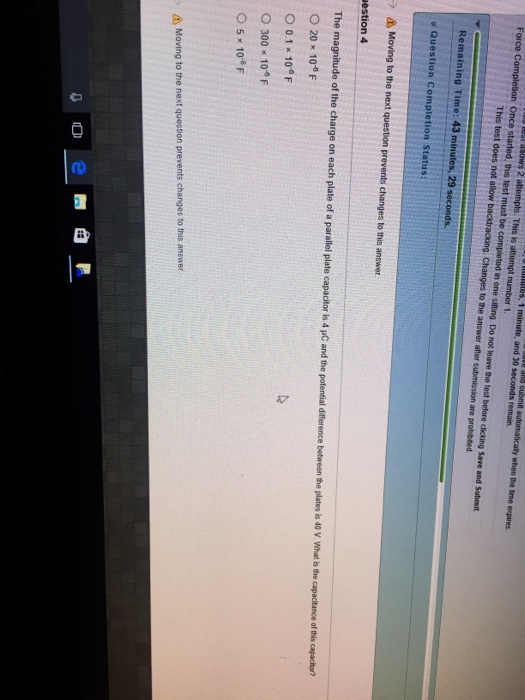The magnitude of the charge on each plate of a parallel plate capacitor is 4 mu C and the potential difference between the plates is 40 V. What is the capacitance of this capacitor? 20 times 10^-0 F 0.1 times 10^-6 F 300 times 10^-6 F 5 times 10^-8 F

• ### A parallel plate air capacitor has a capacitance of C. The charge on each plate is...

A parallel plate air capacitor has a capacitance of C. The charge on each plate is Q. There are no batteries connected to the capacitor. How much work is required to double the separation distance between the plates? Assign values for C (25 μF) and Q (50 μC)

• ### 1. An ideal parallel- plate capacitor has a capacitance of C. If the area of the...

1. An ideal parallel- plate capacitor has a capacitance of C. If the area of the plates is halved and the distance between the plates is doubled, what is the new capacitance? 2. a proton has a. speed of 5.0x10^5 m/s at a point where the electrical potential is 500 V. It moves through a point where the electrical potential is 1000V. What is the speed at this second point? (e=1.60x10^-10C)

• ### Capacitor calculation a) Calculate the capacitance of a parallel-plate capacitor whose plates are made of two...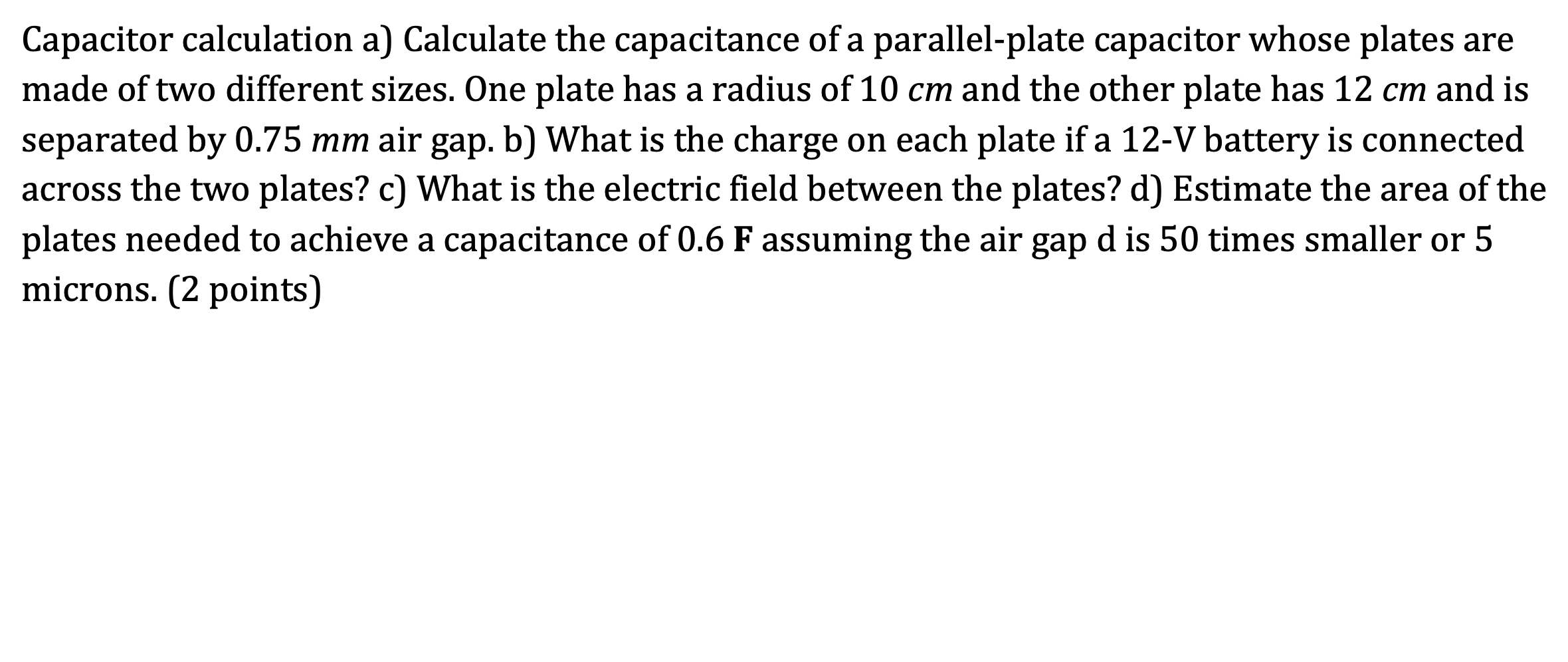Capacitor calculation a) Calculate the capacitance of a parallel-plate capacitor whose plates are made of two different sizes. One plate has a radius of 10 cm and the other plate has 12 cm and is separated by 0.75 mm air gap. b) What is the charge on each plate if a 12-V battery is connected across the two plates? c) What is the electric field between the plates? d) Estimate the area of the plates needed to achieve a capacitance...

• ### . A parallel-plate capacitor of capacitance 100 HF is connected to a power supply of 200...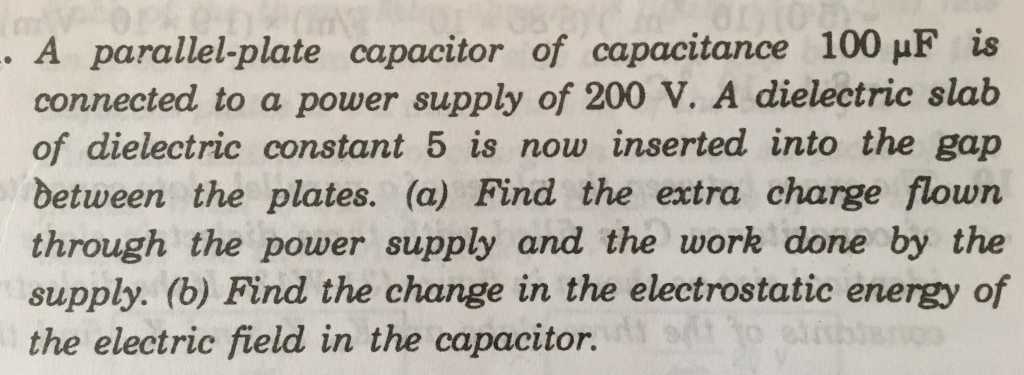. A parallel-plate capacitor of capacitance 100 HF is connected to a power supply of 200 V. A dielectric slab of dielectric constant 5 is now inserted into the gap between the plates. (a) Find the extra charge flown through the power supply and the work done by the supply. (b) Find the change in the electrostatic energy of the electric field in the capacitor.

• ### A parallel plate capacitor has a charge of 7.7 × 10−7 C on one plate and...

A parallel plate capacitor has a charge of 7.7 × 10−7 C on one plate and −7.7 × 10−7 C on the other. The distance between the plates is increased by 22% while the charge on each plate stays the same. What happens to the energy stored in the capacitor? The energy (select) decreases or increases by %.

Free Homework App